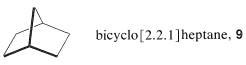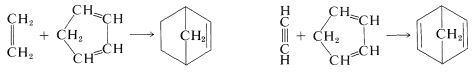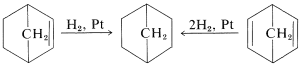# 13.9: Construction of Ring Systems by Cycloaddition

$$\newcommand{\vecs}{\overset { \rightharpoonup} {\mathbf{#1}} }$$ $$\newcommand{\vecd}{\overset{-\!-\!\rightharpoonup}{\vphantom{a}\smash {#1}}}$$$$\newcommand{\id}{\mathrm{id}}$$ $$\newcommand{\Span}{\mathrm{span}}$$ $$\newcommand{\kernel}{\mathrm{null}\,}$$ $$\newcommand{\range}{\mathrm{range}\,}$$ $$\newcommand{\RealPart}{\mathrm{Re}}$$ $$\newcommand{\ImaginaryPart}{\mathrm{Im}}$$ $$\newcommand{\Argument}{\mathrm{Arg}}$$ $$\newcommand{\norm}{\| #1 \|}$$ $$\newcommand{\inner}{\langle #1, #2 \rangle}$$ $$\newcommand{\Span}{\mathrm{span}}$$ $$\newcommand{\id}{\mathrm{id}}$$ $$\newcommand{\Span}{\mathrm{span}}$$ $$\newcommand{\kernel}{\mathrm{null}\,}$$ $$\newcommand{\range}{\mathrm{range}\,}$$ $$\newcommand{\RealPart}{\mathrm{Re}}$$ $$\newcommand{\ImaginaryPart}{\mathrm{Im}}$$ $$\newcommand{\Argument}{\mathrm{Arg}}$$ $$\newcommand{\norm}{\| #1 \|}$$ $$\newcommand{\inner}{\langle #1, #2 \rangle}$$ $$\newcommand{\Span}{\mathrm{span}}$$$$\newcommand{\AA}{\unicode[.8,0]{x212B}}$$

Another example of a synthesis problem makes use of the cycloaddition reactions discussed here. Consider the synthesis of bicyclo[2.2.1]heptane, $$9$$, from compounds with fewer carbons.Whenever a ring has to be constructed, you should consider the possibility of cycloaddition reactions, especially [4 + 2] cycloaddition by the Diels-Alder reaction. A first glance at $$9$$, written in the usual sawhorse-perspective formula, might lead to overlooking the possibility of constructing the skeleton by [4 + 2] addition, because the compound seems only to be made up of five-membered rings. If the structure is rewritten as $$10$$, the six-membered ring stands out much more clearly:If we now try to divide the six-membered ring into  and  fragments, we find that there are only two different ways this can be done:The left division corresponds to a simple [4 + 2] cycloaddition, whereas the right division corresponds to a complex reaction involving formation of three ring bonds at once. Actual Diels-Alder reactions require diene and dienophile starting materials, and two possibilities, using 1,3-cyclopentadiene as the diene and ethene or ethyne as dienophile, follow:Either of the products can be converted to bicyclo[2.2.1]heptane by hydrogenation (Table 13-5):Neither ethene nor ethyne is a very good dienophile but [4 + 2] cycloadditions of either with 1,3-cyclopentadiene go well at temperatures of $$160$$-$$180^\text{o}$$ because 1,3-cyclopentadiene is a very reactive diene. Achieving the overall result of addition of ethene or ethyne to a less reactive diene could necessitate a synthetic sequence wherein one of the reactive dienophiles listed in Table 13-1 is used to introduce the desired two carbons, and the activating groups are subsequently removed. An example follows:Reactions that can be used to remove a $$\ce{-CO_2H}$$ group will be discussed in Chapter 18.